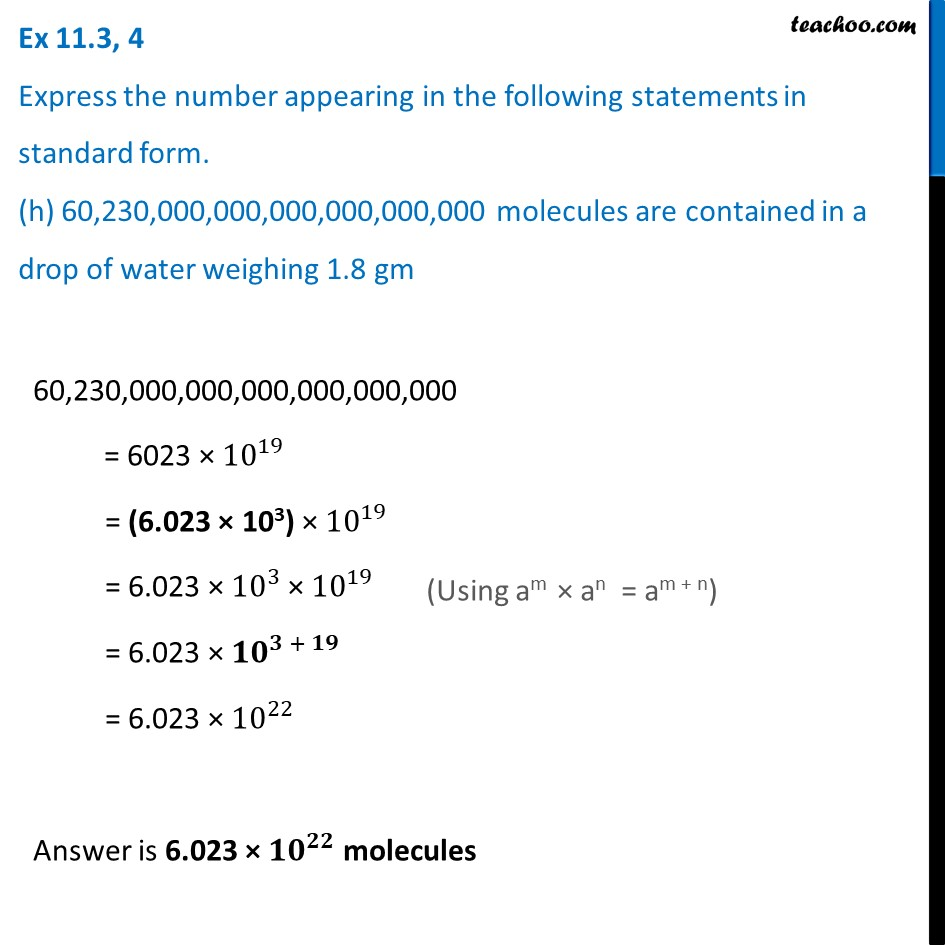Ex 11.3

Chapter 11 Class 7 Exponents and Powers
Serial order wiseLearn in your speed, with individual attention - Teachoo Maths 1-on-1 Class

### Transcript

Ex 11.3, 4 Express the number appearing in the following statements in standard form. (h) 60,230,000,000,000,000,000,000 molecules are contained in a drop of water weighing 1.8 gm 60,230,000,000,000,000,000,000 = 6023 × 〖10〗^19 = (6.023 × 103) × 〖10〗^19 = 6.023 × 〖10〗^3 × 〖10〗^19 = 6.023 × 〖10〗^(3 + 19) = 6.023 × 〖10〗^22 Answer is 6.023 × 〖𝟏𝟎〗^𝟐𝟐 molecules (Using am × an = am + n)